## Minimum-Spanning-Tree

 Question 1

Consider the following undirected graph G:Choose a value for x that will maximize the number of minimum weight spanning trees (MWSTs) of G. The number of MWSTs of G for this value of x is _________.

 A 4 B 5 C 6 D 7
Algorithms       Minimum-Spanning-Tree       GATE 2018       Video-Explanation
Question 1 Explanation:
Here, x = 5 because it is having maximum number of spanning trees.
If x = 5 then the total number of MWSTs are 4.
If r = 1If r = 2If r = 3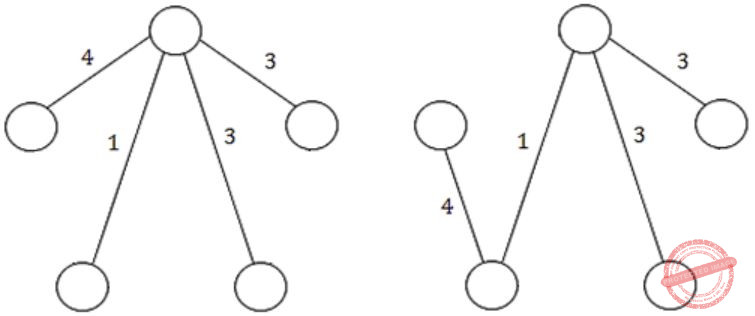If r = 4If r = 5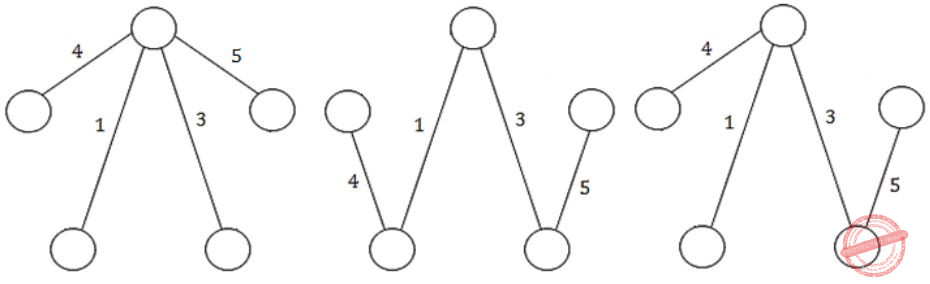Question 2
Let G = (V, E) be any connected undirected edge-weighted graph. The weights of the edges in E are positive and distinct. Consider the following statements:
(I) Minimum Spanning Tree of G is always unique.
(II) Shortest path between any two vertices of G is always unique.
Which of the above statements is/are necessarily true?
 A (I) only B (II) only C both (I) and (II) D neither (I) nor (II)
Algorithms       Minimum-Spanning-Tree       GATE 2017 [Set-1]       Video-Explanation
Question 2 Explanation:
If the graph has all positive and distinct (unique values no duplicates) then Statement-I definitely correct because if we are using either prim’s or kruskal’s algorithm it gives the unique spanning tree.
Let us take an exampleStep 1:
Using kruskal’s algorithm, arrange each weights in ascending order.
17, 18, 20, 21, 22, 23, 24, 25, 26, 27, 28, 29, 30
Step 2:Step 3:
17+18+20+21+22+23+26 = 147
Step 4:
Here, all the elements are distinct. So the possible MCST is 1.
Statement-II: May or may not happen, please take an example graph and try to solve it. This is not correct always.
So, we have to pick most appropriate answer.
 Question 3

Let G be a complete undirected graph on 4 vertices, having 6 edges with weights being 1, 2, 3, 4, 5, and 6. The maximum possible weight that a minimum weight spanning tree of G can have is __________.

 A 7 B 8 C 9 D 10
Algorithms       Minimum-Spanning-Tree       GATE 2016 [Set-1]       Video-Explanation
Question 3 Explanation:
Let G be a complete undirected graph with 4 vertices & 6 edges so according to graph theory, if we use Prim’s / Kruskal’s algorithm, the graph looks like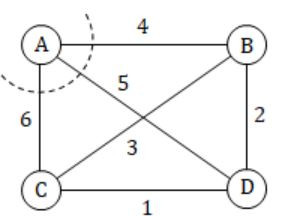Now consider vertex A to make Minimum spanning tree with Maximum weights.
As weights are 1, 2, 3, 4, 5, 6. AB, AD, AC takes maximum weights 4, 5, 6 respectively.
Next consider vertex B, BA = 4, and minimum spanning tree with maximum weight next is BD & BC takes 2, 3 respectively.
And the last edge CD takes 1.
So, 1+2+4 in our graph will be the Minimum spanning tree with maximum weights.
 Question 4
G = (V,E) is an undirected simple graph in which each edge has a distinct weight, and e is a particular edge of G. Which of the following statements about the minimum spanning trees (MSTs) of G is/are TRUE?
I. If e is the lightest edge of some cycle in G, then every MST of G includes e
II. If e is the heaviest edge of some cycle in G, then every MST of G excludes e
 A I only B II only C both I and II D neither I nor II
Algorithms       Minimum-Spanning-Tree       GATE 2016 [Set-1]       Video-Explanation
Question 4 Explanation:
Statement-1: False
The MSTs of G may or may not include the lightest edge.
Take rectangular graph labelled with P,Q,R,S.
Connect with P-Q = 5, Q-R = 6, R-S = 8, S-P = 9, P-R = 7.
When we are forming a cycle R-S-P-R. P-R is the lightest edge of the cycle.
The MST abcd with cost 11
P-Q + Q-R + R-S does not include it.
Statement-2: True
Suppose there is a minimum spanning tree which contains e. If we add one more edge to the spanning tree we will create a cycle.
Suppose we add edge e to the spanning tree which generated cycle C.
We can reduce the cost of the minimum spanning tree if we choose an edge other than e from C for removal which implies that e must not be in minimum spanning tree and we get a contradiction.
 Question 5

The graph shown below 8 edges with distinct integer edge weights. The minimum spanning tree (MST) is of weight 36 and contains the edges: {(A, C), (B, C), (B, E), (E, F), (D, F)}. The edge weights of only those edges which are in the MST are given in the figure shown below. The minimum possible sum of weights of all 8 edges of this graph is ______________.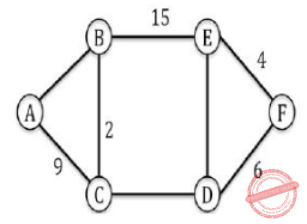A 69 B 70 C 71 D 72
Algorithms       Minimum-Spanning-Tree       GATE 2015 [Set-1]
Question 5 Explanation: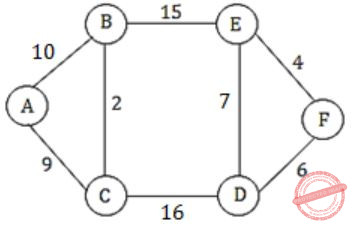⇒ Total sum = 10 + 9 + 2 + 15 + 7 + 16 + 4 + 6 = 69

--> First we compare A-C and A-B we find 9 at A-C it means A-B must greater than A-C and for minimum possible greater value than 9 will be 10
-> Second we compare B-E and C-D in which we select B-E is 15 which C-D possible weight 16.
-> Third, we compare E-D and F-D in which we select F-D 6 means E-D must be greater than 6 so possible value greater than 6 is 7 .
 Question 6

Let G be a connected undirected graph of 100 vertices and 300 edges. The weight of a minimum spanning tree of G is 500. When the weight of each edge of G is increased by five, the weight of a minimum spanning tree becomes __________.

 A 995 B 996 C 997 D 998
Algorithms       Minimum-Spanning-Tree       GATE 2015 [Set-3]
Question 6 Explanation:
G has 100 vertices ⇒ spanning tree contain 99 edges given, weight of a minimum spanning tree of G is 500 since, each edge of G is increased by five. ∴ Weight of a minimum spanning tree becomes 500 + 5 ⨯ 99= 995
 Question 7

The number of distinct minimum spanning trees for the weighted graph below is _______.A 6 B 7 C 8 D 9
Algorithms       Minimum-Spanning-Tree       GATE 2014 [Set-2]
Question 7 Explanation:Minimum Spanning Tree:From the diagram, CFDA gives the minimum weight so will not disturb them, but in order to reach BE=1 we have 3 different ways ABE/ DBE/ DEB and we have HI=1, the shortest weight, we can reach HI=1 through GHI/ GIH.
So 3*2=6 ways of forming Minimum Spanning Tree with sum as 11.
 Question 8

Let G be a weighted graph with edge weights greater than one and G' be the graph constructed by squaring the weights of edges in G. Let T and T' be the minimum spanning trees of G and G', respectively, with total weights t and t'. Which of the following statements is TRUE?

 A T' = T with total weight t' = t2 B T' = T with total weight t'2 C T' ≠ T but total weight t' = t2 D None of the above
Algorithms        Minimum-Spanning-Tree       GATE 2012
Question 8 Explanation:
Let graph G beThen MST for G is,Now let's square the weights,Then MST for G' is,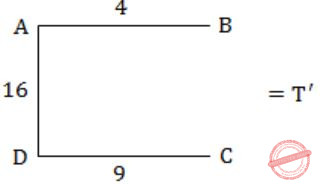So, from above we can see that T is not necessarily equal to T' and moreover (t1) < (t2).
So option (D) is correct answer.
 Question 9

An undirected graph G(V, E) contains n (n > 2) nodes named v1, v2, ….vn. Two nodes vi , vj are connected if and only if 0 < |i – j| ≤ 2. Each edge (vi, vj) is assigned a weight i + j. A sample graph with n = 4 is shown below.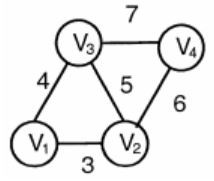What will be the cost of the minimum spanning tree (MST) of such a graph with n nodes?

 A 1/12(11n2-5n) B n2 – n + 1 C 6n – 11 D 2n + 1
Algorithms        Minimum-Spanning-Tree       GATE 2011
Question 9 Explanation:
Let take example of 5 vertices,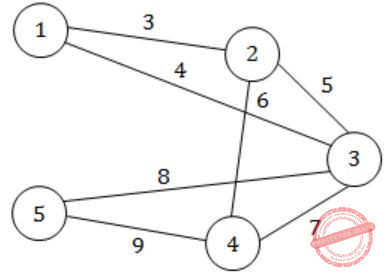Cost of MST,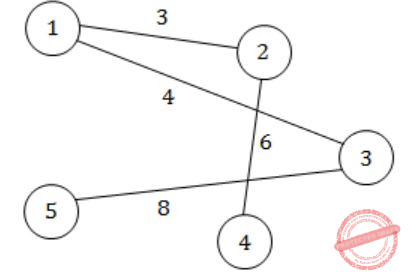= 3+4+6+8 = 21
Only option (B) satisfies it.
 Question 10

An undirected graph G(V, E) contains n (n > 2) nodes named v1, v2, ….vn. Two nodes vi , vj are connected if and only if 0 < |i – j| ≤ 2. Each edge (vi, vj) is assigned a weight i + j. A sample graph with n = 4 is shown below.The length of the path from v5 to v6 in the MST of previous question with n  =10 is

 A 11 B 25 C 31 D 41
Algorithms        Minimum-Spanning-Tree       GATE 2011
Question 10 Explanation:
Lets first draw graph with 10 vertices,Now MST of above graph is,∴ The length of path from v5 to v6 in the MST is,
8+4+3+6+10 = 31
 Question 11

Consider a complete undirected graph with vertex set {0, 1, 2, 3, 4}. Entry Wij in the matrix W below is the weight of the edge {i, j}.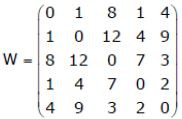What is the minimum possible weight of a spanning tree T in this graph such that vertex 0 is a leaf node in the tree T?

 A 7 B 8 C 9 D 10
Algorithms        Minimum-Spanning-Tree       GATE 2010
Question 11 Explanation:
Here the point to be noted is that vertex 0 is a leaf node. So degree of vertex 0 cannot be more than or equal to 2.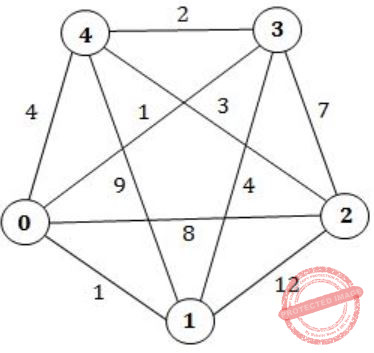So, the edges of the spanning tree given that vertex 0 is a leaf node in the tree,So, the minimum possible weight of spanning tree will be
= 1 + 4 + 2 + 3
= 10
 Question 12

Consider the following graph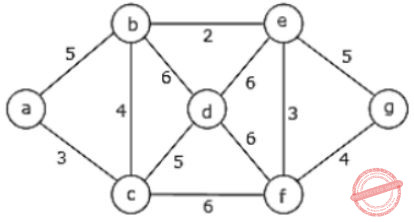Which one of the following is NOT the sequence of edges added to the minimum spanning tree using Kruskal’s algorithm?

 A (b,e)(e,f)(a,c)(b,c)(f,g)(c,d) B (b,e)(e,f)(a,c)(f,g)(b,c)(c,d) C (b,e)(a,c)(e,f)(b,c)(f,g)(c,d) D (b,e)(e,f)(b,c)(a,c)(f,g)(c,d)
Algorithms        Minimum-Spanning-Tree       GATE 2009
Question 12 Explanation:
To find Minimum Spanning Tree(MST) using Kruskal’s algorithm we have to follow standard procedure is
1. It follows like forest structure(Means we are disconnecting all the edges connected to vertices).
2. Sort edge weights in Ascending order.
3. Always select the minimum edge weight first according to Spanning Tree rules.
As per the options below Option A,B,C, are following correct order but Option D is violating Kruskal’s procedure. The edge between a-c of weight 4 comes after b-c of weight 3.
 Question 13

Let w be the minimum weight among all edge weights in an undirected connected graph. Let e be a specific edge of weight w. Which of the following is FALSE?

 A There is a minimum spanning tree containing e. B If e is not in a minimum spanning tree T, then in the cycle formed by adding e to T, all edges have the same weight. C Every minimum spanning tree has an edge of weight w. D e is present in every minimum spanning tree.
Algorithms        Minimum-Spanning-Tree       GATE 2007
Question 13 Explanation:
To find minimum Spanning tree(MST), may not be present ‘e’ in every MST graph.
 Question 14
Consider a weighted complete graph G on the vertex set {v1, v2, ..., vn} such that the weight of the edge (vi, vj) is 2|i - j|. The weight of a minimum spanning tree of G is:
 A n-1 B 2n-2 CD n2
Algorithms        Minimum-Spanning-Tree       GATE 2006
Question 14 Explanation:
1) Including the initial call which means write the length of the spanning tree, a simple one it is. (Based on a particular graph)
2) Weight of the minimum spanning tree = 2|2 - 1| + 2|3 - 2| + 2|4 - 3| + 2|5 - 4| .... + 2| n - (n-1) | = 2n - 2
 Question 15

Consider the following graph:Which one of the following cannot be the sequence of edges added, in that order, to a minimum spanning tree using Kruskal’s algorithm?

 A (a—b),(d—f),(b—f),(d—c),(d—e) B (a—b),(d—f),(d—c),(b—f),(d—e) C (d—f),(a—b),(d—c),(b—f),(d—e) D (d—f),(a—b),(b—f),(d—e),(d—c)
Algorithms        Minimum-Spanning-Tree       GATE 2006
Question 15 Explanation:
To find Minimum Spanning Tree(MST) using Kruskal’s algorithm we have to follow standard procedure is
1. It follows like forest structure (Means we are disconnecting all the edges connected to vertices).
2. Sort edge weights in Ascending order.
3. Always select the minimum edge weight first according to Spanning Tree rules.
Note: The edge d-e cannot be considered before d-c.
 Question 16

What is the weight of a minimum spanning tree of the following graph ?A 29 B 31 C 38 D 41
Algorithms        Minimum-Spanning-Tree       GATE 2003
Question 16 Explanation:1+2+3+2+8+4+4+2+5 =31
 Question 17

Consider a weighted undirected graph with vertex set V = {n1,n2,n3,n4,n5,n6} and edge set

``` E = {(n1,n2,2),(n1,n3,8),(n1,n6,3),(n2,n4,4),(n2,n5,12),(n3,n4,7),(n4,n5,9),
(n4,n6,4)}. The third value in each tuple represents the weight of the edge
specified in the tuple. ```

(a) List the edges of a minimum spanning tree of the graph.
(b) How many distinct minimum spanning trees does this graph have?
(c) Is the minimum among the edge weights of a minimum spanning tree unique overall possible minimum spanning trees of a graph?
(d) Is the maximum among the edge weights of a minimum spanning tree unique over all possible minimum spanning trees of a graph?

 A Theory Explanation is given below.
Algorithms        Minimum-Spanning-Tree       GATE 2001
Question 17 Explanation:
(b) 2 distinct MST's.
(c) Yes, it always. Because 'the edge weight 2 is unique'.
(d) Yes, it always. Because 'the edge weight 9 is unique'.
 Question 18

Let G be an undirected connected graph with distinct edge weight. Let emax be the edge with maximum weight and  emin the edge with minimum weight. Which of the following statements is false?

 A Every minimum spanning tree of G must contain emin B If emax is in a minimum spanning tree, then its removal must disconnect G C No minimum spanning tree contains emax D G has a unique minimum spanning tree
Algorithms        Minimum-Spanning-Tree       GATE 2000
Question 18 Explanation:Minimum Spanning Tree:Question 19

A complete, undirected, weighted graph G is given on the vertex {0, 1,...., n−1} for any fixed ‘n’. Draw the minimum spanning tree of G if
(a) the weight of the edge (u,v) is ∣u − v∣
(b) the weight of the edge (u,v) is u + v

 A Theory Explanation.
Algorithms       Minimum-Spanning-Tree       GATE 1996
 Question 20

How many minimum spanning trees does the following graph have? Draw them. (Weights are assigned to the edge).A Theory Explanation.
Algorithms       Minimum-Spanning-Tree       GATE 1995
 Question 21

Let G = (V,E) be a weighted undirected graph and let T be a Minimum Spanning Tree (MST) of G maintained using adjacency lists. Suppose a new weighted edge (u,v) ∈ V×V is added to G. The worst case time complexity of determining if T is still an MST of the resultant graph is

 A θ(|E|+|V|) B θ(|E| log|V|) C θ(|E||V|) D θ(|V|)
Algorithms       Minimum-Spanning-Tree       GATE 2020
Question 21 Explanation:
Method-1:
• As T is a minimum spanning tree and we need to add a new edge to existing spanning tree.
• Later we need to check still T is a minimum spanning tree or not, So we need to check all vertices whether there is any cycle present after adding a new edge.
• All vertices need to traverse to confirm minimum spanning tree after adding new edge then time complexity is O(V).
Method-2:
Time Complexity:
Total vertices: V, Total Edges : E
• O(logV) – to extract each vertex from the queue. So for V vertices – O(VlogV)
• O(logV) – each time a new pair object with a new key value of a vertex and will be done at most once for each edge. So for total E edge – O(ElogV)
• So overall complexity: O(VlogV) + O(ElogV) = O((E+V)logV) = O(ElogV)
Note: Method-1 is the most appropriate answer for giving a question.
 Question 22

Consider a graph G = (V, E), where V = {v1, v2, …, v100}, E = {(vi, vj) | 1 ≤ i < j ≤ 100}, and weight of the edge (vi, vj) is |i - j|. The weight of the minimum spanning tree of G is ______.

 A 99
Algorithms       Minimum-Spanning-Tree       GATE 2020
Question 22 Explanation:
• If there are n vertices in the graph, then each spanning tree has n − 1 edges.
• N =100
• Edge weight is |i-j| for Edge (vi,vj) {1<=i<=100}
• The weight of edge(v1,v2) is 1 , edge(v5,v6) is 1.
• So, 99 edges of weight is 99.
 Question 23

For the undirected, weighted graph given below, which of the following sequences of edges represents a correct execution of Prim's algorithm to construct a Minimum Span­ning Tree?A (a, b), (d, f), (f, c), (g, i), (d, a), (g, h), (c, e), (f, h) B (c, e), (c, f), (f, d), (d, a), (a, b), (g, h), (h, f), (g, i) C (d, f), (f, c), (d, a), (a, b), (c, e), (f, h), (g, h), (g, i) D (h, g), (g, i), (h, f), (f, c), (f, d), (d, a), (a, b), (c, e)
Algorithms        Minimum-Spanning-Tree       GATE 2008-IT
Question 23 Explanation:
Prim's algorithm starts from any vertex and expands MST by adding one vertex in each step which is close to the intermediate MST (made till previous step).
(A) (d, f) is chosen but neither 'd' nor 'f' vertices are part of previous MST (MST made till previous step).
(B) (g, h) is chosen but neither g or h vertices are part of MST made till previous step.
(C) Correct.
(D) (f, c) is chosen, but at that point (f, d) had less weight. So, (f , d) should have been chosen.
 Question 24

What is the largest integer m such that every simple connected graph with n vertices and n edges contains at least m different spanning trees?

 A 1 B 2 C 3 D n
Algorithms        Minimum-Spanning-Tree       GATE 2007-IT
Question 24 Explanation:
If graph is connected and contains n vertices and n edges means there is possibility of only one cycle.
→ If we create a different spanning tree by removing one edge at a time.
→ For cycle required 3 minimum edges. So there is at least 3 spanning trees in such a graph.
 Question 25
Consider the following undirected graph with edge weights as shown:The number of minimum-weight spanning trees of the graph is _______
 A 3
Algorithms       Minimum-Spanning-Tree       GATE 2021 CS-Set-1
Question 25 Explanation:

To find the number of spanning trees using 2 methods:

1. If graph is complete, use n^n-2 formula
2. If graph is not complete, then use kirchhoff theorem.

Steps in Kirchoff’s Approach:

(i) Make an Adjacency matrix.

(ii) Replace all non-diagonal is by – 1.

(iii) Replace all diagonal zero’s by the degree of the corresponding vertex.

(iv) Co-factors of any element will give the number of spanning trees.

Using the Kirchhoff theorem will take lot of time because the number of vertices are 9.

So, we use brute force technique to solve the problem with the help of Kruskal's algorithm.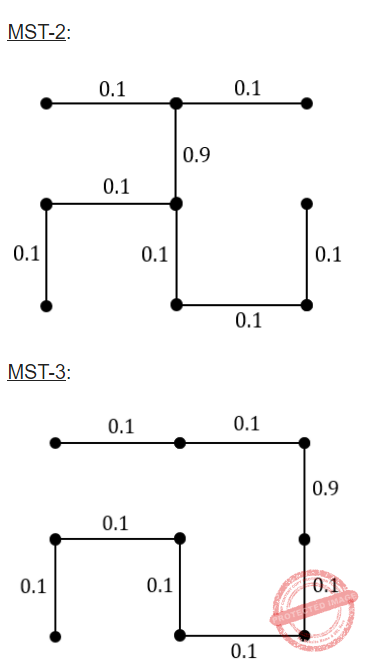Question 26
Let G be a connected undirected weighted graph. Consider the following two statements.
S1: There exists a minimum weighted edge in G which is present in every minimum spanning tree of G.
S2:If every edge in G has distinct weight, then G has a unique minimum spanning tree.
Which of the following options is correct?
 A S1is false and S2is true. B S1is true and S2is false. C Both S1 and S2are false. D Both S1 and S2are true.
Algorithms       Minimum-Spanning-Tree       GATE 2021 CS-Set-2
Question 26 Explanation:

Statement-1: FALSE: The given statement is not valid for all the cases because they are not mentioned, edge weights are in distinct or duplicate. So, we can take any random edge weights for the given statement.

Example:Statement-2: TRUE: Using the kruskal’s (or) prim's algorithm we get a unique MST when there is a unique edge weight.

Example:Based on the above graph, we get the MST isQuestion 27
In a directed acyclic graph with a source vertex s, the quality-score of s directed path is defined to be the product of the weights of the edges on the path. Further, for a vertex v other than s, the quality-score of v is defined to be the maximum among the quality-scores of all the paths from s to v. The quality-score of s is assumed to be 1.The sum of the quality-scores of all the vertices in the graph shown above is _________.
 A 929
Algorithms       Minimum-Spanning-Tree       GATE 2021 CS-Set-2
 Question 28
Consider a simple undirected weighted graph G , all of whose edge weights are distinct. Which of the following statements about the minimum spanning trees of G is/are TRUE?
 A The edge with the second smallest weight is always part of any minimum spanning tree of G . B One or both of the edges with the third smallest and the fourth smallest weights are part of any minimum spanning tree of G . CD G can have multiple minimum spanning trees.
Algorithms       Minimum-Spanning-Tree       GATE 2022
Question 28 Explanation:
Let assume the graph and minimum spanning tree of the corresponding graph.Option-A: TRUE: As per the above graph, the second minimum edge weight is also part of the MST.
The second smallest weight is always in MST because it will not form a cycle.
Option-B: FALSE: As per the above graph, the third minimum edge weight is not part of the MST.
The third or fourth edge weight may be part of the cycle. So, it may or may not be in MST.
Option-C: TRUE: As per the example graph, it is always correct.
Option-D: FALSE: We will get a unique minimum spanning tree if edge weights are distinct.
 Question 29
Let G ( V , E ) be a directed graph, where V = {1, 2,3, 4, 5} is the set of vertices and E is the set of directed edges, as defined by the following adjacency matrix A .A 24
Algorithms       Minimum-Spanning-Tree       GATE 2022
Question 29 Explanation:
Graph have 2 elements --> We get 1 MST
Graph have 3 elements --> We get 2 MSTs
Graph have 4 elements --> We get 6 MSTs (3*2*1)
Graph have 5 elements --> We get 24 MSTs(4*3*2*1)
 Question 30

Consider the graph shown below :Use Kruskal’s algorithm to find the minimum spanning tree of the graph. The weight of this minimum spanning tree is

 A 13 B 16 C 17 D 14
Algorithms       Minimum-spanning-Tree       UGC-NET CS 2018 DEC Paper-2
Question 30 Explanation:The weight of this minimum spanning tree is 16.
 Question 31
Let G be an undirected connected graph with distinct edge weight. Let Emax be the edge with maximum weight and E​ min​ the edge with minimum weight. Which of the following statements is false?
 A Every minimum spanning tree of G must contain E​ min. B If E​ max​ is in minimum spanning tree, then its removal must disconnect G. C No minimum spanning tree contains E​ max. D G has a unique minimum spanning tree.
Algorithms       Minimum-Spanning-Tree       UGC NET CS 2017 Nov- paper-2
Question 31 Explanation:Minimum Spanning Tree: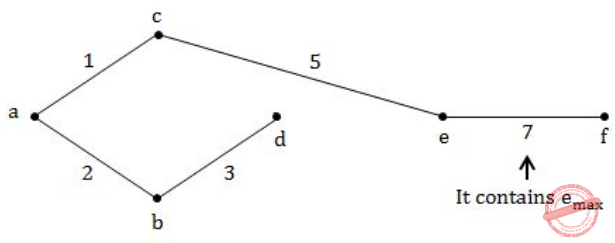Question 32A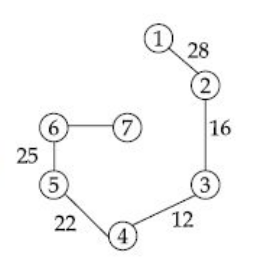BC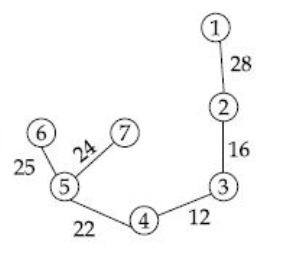D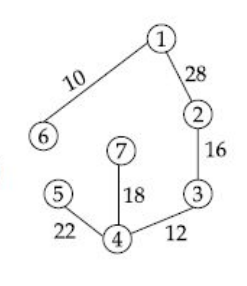Algorithms       Minimum-Spanning-Tree       UGC NET CS 2015 Jun- paper-2
Question 32 Explanation:
To find minimum cost spanning tree, we are using either prim’s algorithm (or) kruskal’s algorithm.
According to kruskal’s algorithm, first we have sort an elements.
Elements are: 10,12,14,16,18,22,24,25,28Question 33
Which of the following connected simple graph has exactly one spanning tree ?
 A Complete graph B Hamiltonian graph C Euler graph D None of the above
Algorithms       Minimum-Spanning-Tree       UGC NET CS 2013 June-paper-2
Question 33 Explanation:
→ Complete graph have nn-2 spanning trees.
→ Hamiltonian graph will get more than one spanning tree.
→ Euler graph will get more than one spanning tree.
 Question 34
The total number of spanning trees that can be drawn using five labelled vertices is :
 A 125 B 64 C 36 D 16
Algorithms       Minimum-Spanning-Tree       UGC NET CS 2008 Dec-Paper-2
Question 34 Explanation:
To find total number of spanning trees we are using a standard formula is nn-2.
n=5
=55-2
=53
=125
 Question 35
The complexity of Kruskal’s minimum spanning tree algorithm on a graph with ‘n’ nodes and ‘e ’ edges is :
 A O (n) B O (n log n) C O (e log n) D O (e)
Algorithms       Minimum-Spanning-Tree       UGC NET CS 2008-june-Paper-2
Question 35 Explanation:
MST-KRUSKAL(G,w)
1. A=∅
2. for each vertex v∈ G.V
3. MAKE-SET(v)
4. sort the edges of G.E into nondecreasing order by weight w
5. for each edge (u,v)∈G.E, taken in nondecreasing order by weight
6. if FIND-SET(u)≠FIND-SET(v)
7. A=A∪{(u,v)}
8. UNION(u,v)
9. return A
Analysis:
The running time of Kruskal’s algorithm for a graph G=(V,E) depends on how we implement the disjoint-set data structure.
→ Initializing the set A in line 1 takes O(1) time, and the time to sort the edges in line 4 is O(ElgE). (We will account for the cost of the |V| MAKE-SET operations in the for loop of lines 2–3 in a moment.)
→ The for loop of lines 5–8 performs O(E) FIND-SET and UNION operations on the disjoint-set forest.
→ Along with the |V| MAKE-SET operations, these take a total of O((V+E)α(V)) time, where α is the very slowly growing function.
→ Because we assume that G is connected, we have |E|>=|V|-1, and so the disjoint-set operations take O(Eα(V)) time.
→ Moreover, since α |V|=O(lg V)=O(lg E), the total running time of Kruskal’s algorithm is O(ElgE)
→ Observing that |E|<|V|2 , we have lg |E|=O(lg V), and so we can restate the running time of Kruskal’s algorithm as O(E lg V)
 Question 36
An undirected graph G (V, E) contains n (n > 2) nodes named v1 , v2 ,...,vn. Two nodes vi and vj are connected if and only if 0 <│i−j│≤2. Each edge (vi , vj ) is assigned a weight i+j. The cost of the minimum spanning tree of such a graph with 10 nodes is:
 A 88 B 91 C 49 D 21
Algorithms       Minimum-Spanning-Tree       UGC NET CS 2017 Nov- paper-3
Question 36 Explanation:
Method-1: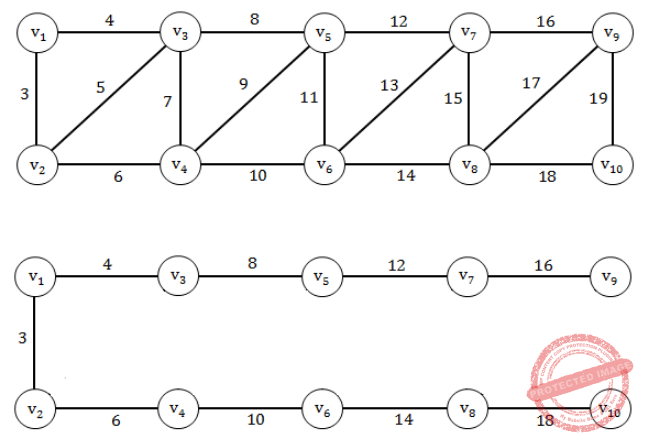Question 37
Consider a weighted complete graph G on the vertex set {ν1, ν2, .... νn} such that the weight of the edge (νi, νj) is 4 | i – j|. The weight of minimum cost spanning tree of G is :
 A 4n2 B n C 4n-4 D 2n-2
Algorithms       Minimum-Spanning-Tree       UGC NET CS 2016 Aug- paper-3
Question 37 Explanation:
1) Including the initial call which means write the length of the spanning tree, a simple one it is. (Based on a particular graph)
2)Weight of the minimum spanning tree
= 4|2 - 1| + 4|3 - 2| + 4|4 - 3| + 4|5 - 4| .... + 4| n - (n-1) |
= 4n - 4
 Question 38
How many distinct minimum weight spanning trees does the following undirected, weighted graph have?A 1 B 2 C 4 D 6 E 8
Algorithms       Minimum-Spanning-Tree       TIFR PHD CS & SS 2018
 Question 39
Let n ≥ 3, and let G be a simple, connected, undirected graph with the same number n of vertices and edges. Each edge of G has a distinct real weight associated with it. Let T be the minimum weight spanning tree of G. Which of the following statements
is NOT ALWAYS TRUE?
 A The minimum weight edge of G is in T. B The maximum weight edge of G is not in T. C G has a unique cycle C and the minimum weight edge of C is also in T. D G has a unique cycle C and the maximum weight edge of C is not in T. E T can be found in O(n) time from the adjacency list representation of G.
Algorithms       Minimum-Spanning-Tree       TIFR PHD CS & SS 2018
 Question 40
How many distinct minimum weight spanning trees does the following undirected, weighted graph have?A 8 B 16 C 32 D 64 E None of the above
Algorithms       Minimum-Spanning-Tree       TIFR PHD CS & SS 2019
 Question 41
Consider the following undirected connected graph G with weights on its edges as given in the figure below. A minimum spanning tree is a spanning tree of least weight and a maximum spanning tree is one with largest weight. A second-best minimum spanning tree is a spanning tree whose weight is the smallest among all spanning trees that are not minimum spanning trees in G.Which of the following statements is TRUE in the above graph? (Note that all the edge weights are distinct in the above graph)
 A There is more than one minimum spanning tree and similarly, there is more than one maximum spanning tree here. B There is a unique minimum spanning tree, however there is more than one maximum spanning tree here. C There is more than one minimum spanning tree, however there is a unique maximum spanning tree here. D There is more than one minimum spanning tree and similarly, there is more than one second-best minimum spanning tree here. E There is a unique minimum spanning tree, however there is more than one second-best minimum spanning tree here.
Algorithms       Minimum-Spanning-Tree       TIFR PHD CS & SS 2015
 Question 42
Consider the following undirected graph with some edge costs missingSuppose the wavy edges form a Minimum Cost Spanning Tree for G. Then, which of the following inequalities NEED NOT hold?
 A cost(a, b) ≥ 6. B cost(b, e) ≥ 5 C cost(e, f) ≥ 5. D cost(a, d) ≥ 4 E cost(b, c) ≥ 4
Algorithms       Minimum-Spanning-Tree       TIFR PHD CS & SS 2014
 Question 43
Let G = (V,E) be an undirected connected simple (i.e., no parallel edges or self-loops) graph with the weight function w : E → R on its edge set. Let w(e1) < w(e2) < ··· < w(em), where E = {e1, e2,...,em}. Suppose T is a minimum spanning tree of G.
Which of the following statements is FALSE?
 A The tree T has to contain the edge e1 B The tree T has to contain the edge e2. C The minimum weight edge incident on each vertex has to be present in T D T is the unique minimum spanning tree in G. E If we replace each edge weight wi = w(ei) by its square w2i , then T must still be a minimum spanning tree of this new instance.
Algorithms       Minimum-Spanning-Tree       TIFR PHD CS & SS 2014
 Question 44
Consider an undirected weighted complete graph G with 2016 vertices listed in a set {v1,v2,,..., v2016} such that the weight on edge (vi, vj) is 2|i-j| The weight of a minimum spanning tree of G is
 A 2015 B 4030 C 1008 D None of the above
Algorithms       Minimum-Spanning-Tree       HCU PHD CS MAY 2016
Question 44 Explanation:
The minimum weight that a edge can have inthe graph given above is 2.And every adjacent vertices numerically wil have weight 2.And we know that MST contains n-1 edges,where n=2016.
So weight of MST is (n-1)*2 = (2016-1)*2=4030
 Question 45
Consider the undirected graph below: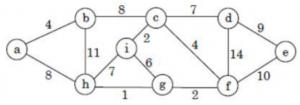Using Prim’s algorithm to construct a minimum spanning tree starting with node a, which one of the following sequences of edges represents a possible order in which the edges would be added to construct the minimum spanning tree?
 A (a,b), (a,h), (g,h), (f,g), (c,f), (c,i), (c,d), (d,e) B (a,b), (b,h), (g,h), (g,i), (c,i), (c,f), (c,d), (d,e) C (a,b), (b,c), (c,i), (c,f), (f,g), (g,h), (c,d), (d,e) D (a,b), (g,h), (g,f), (c,f), (c,i), (f,e), (b,c), (d,e) E A and C
Algorithms       Minimum-Spanning-Tree       UGC NET JRF November 2020 Paper-2
Question 45 Explanation:The final sequence is a-b, b-c, c-i, c-f, f-g, g-h, c-d, d-e
There are 45 questions to complete.